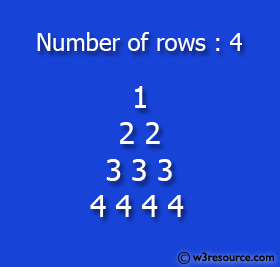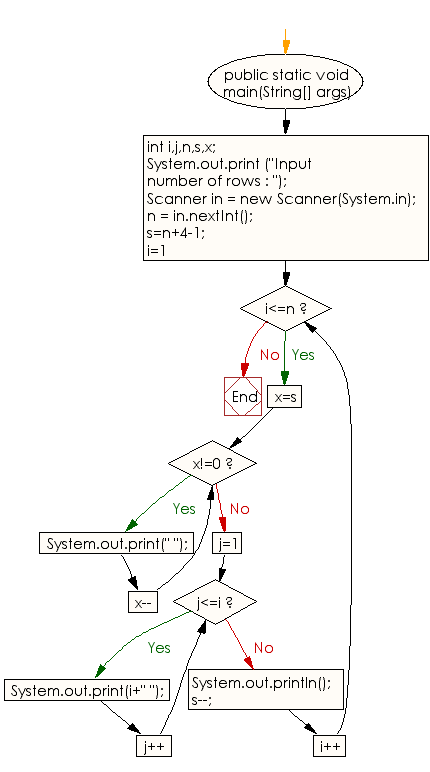﻿ Java exercises: Print a pattern like a pyramid with a number which will repeat the number in the same row - w3resource

# Java Conditional Statement Exercises: Print a pattern like a pyramid

## Java Conditional Statement: Exercise-19 with Solution

Write a program in Java to print such pattern like a pyramid with a number which will repeat the number in the same row.

```       1
2 2
3 3 3
4 4 4 4
```

Test Data
Input number of rows : 4

Pictorial Presentation:Sample Solution:

Java Code:

``````import java.util.Scanner;
public class Exercise19 {

public static void main(String[] args)
{
int i,j,n,s,x;
System.out.print ("Input number of rows : ");
Scanner in = new Scanner(System.in);
n = in.nextInt();

s=n+4-1;
for(i=1;i<=n;i++)
{
for(x=s;x!=0;x--)
{
System.out.print(" ");
}
for(j=1;j<=i;j++)
{
System.out.print(i+" ");
}
System.out.println();
s--;
}
}
}
```
```

Sample Output:

```Input number of rows : 4
1
2 2
3 3 3
4 4 4 4
```

Flowchart:Java Code Editor: